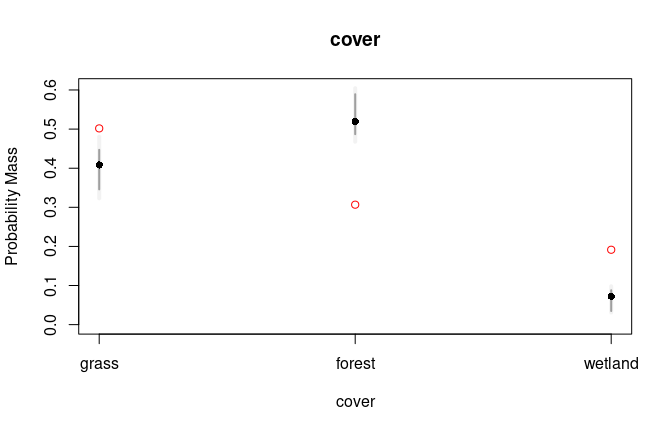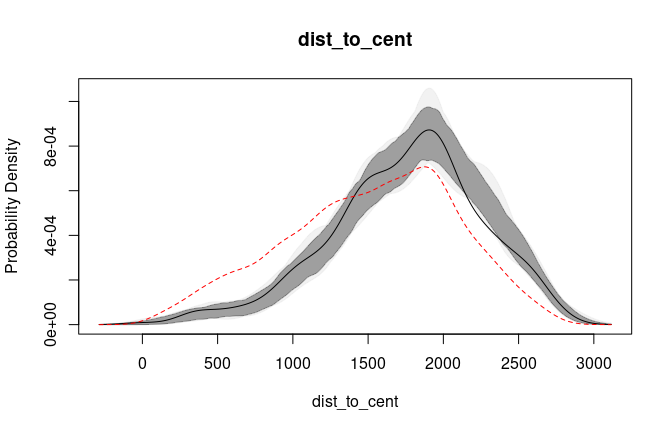# UHC Plots

## Background

Used-habitat calibration plots (UHC plots) are a graphical method for evaluating the calibration of a fitted HSF or (i)SSF model. The method uses a combination of parametric (to account for parameter uncertainty) and empirical bootstrapping to identify the distribution of used habitat, conditional on the fitted model and a test dataset. It can be used to identify problems in model specification, such as missing predictors and misspecified functional forms (e.g., linear rather than quadratic terms). For more details, see Fieberg et al. (2018).

## Implementation in `amt`

We currently have an implementation of UHC plots in `amt` for objects of class `fit_logit` and `glm` (HSFs) and `fit_clogit` (iSSFs). The approach for both models is similar, making use of generic functions with appropriate methods depending on the class.

### General workflow

For either an HSF or (i)SSF, the workflow is as follows:

1. Prepare data for analysis
2. Split data into training and testing datasets.
3. Use training dataset to fit candidate model(s) [HSF or (i)SSF].
4. Prepare UHC plots by passing the fitted model and the testing data to the function `prep_uhc()`.
5. Create the UHC plots by calling `plot()` on the object returned in (4).

## Examples

`amt` contains simulated habitat data. This was once stored as a `RasterStack`, but with conversion from `raster` to `terra`, it is no longer possible to store a `SpatRaster` as `*.rda` (the default binary format for included data). Instead, `uhc_dat` is a `data.frame` that can be converted to a `SpatRaster` with `terra::rast()`. For example:

``hab <- rast(uhc_dat, type = "xyz", crs = "epsg:32612")``

`amt` also contains location data simulated under (1) an HSF and (2) an iSSF to demonstrate the UHC plot workflow.

The `SpatRaster` (`hab`, once converted) contains 7 layers:

• `forage`: forage biomass in g/m2 (a resource)
• `temp`: mean annual temperature in °C (a condition)
• `pred`: predator density in predators/100 km2 (a risk)
• `cover`: land cover categories (1 = grassland, 2 = forest, 3 = wetland)
• `dist_to_water`: distance to the wetland category in m (does not affect selection)
• `dist_to_cent`: distance to the center of the raster in m (does not affect selection in HSF; avoidance of large distance to center in iSSF)
• `rand`: random integer from -4 to 4 (does not affect selection)

Note that `temp`, a condition, is modeled with a quadratic term in the simulations.

True parameter values under the simulation are in the description for each dataset, i.e., `?uhc_hsf_locs` and `?uhc_issf_locs`.

``````# Load all the packages we need
library(amt)
library(dplyr)
library(terra)
library(sf)

data(uhc_hab)
hab <- rast(uhc_hab, type = "xyz", crs = "epsg:32612")
# Convert "cover" layer to factor
levels(hab[]) <- data.frame(id = 1:3,
cover = c("grass", "forest", "wetland"))

# Affect habitat selection in simulation
plot(hab[[1:4]])``````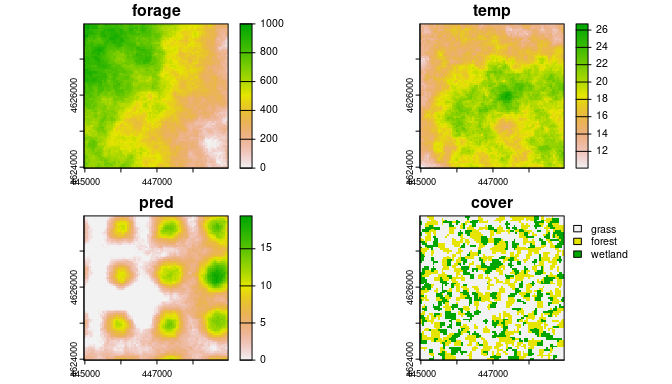``````
# Do not affect habitat selection in simulation
plot(hab[[5:7]])``````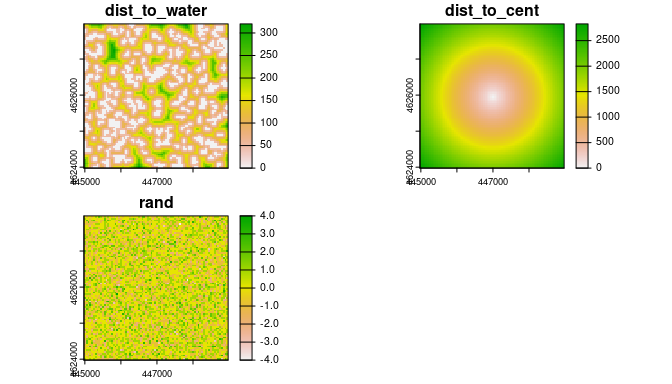### HSF Example

The following is a demonstration of the workflow for an HSF.

#### Prepare data

First, we combine steps (1) and (2) by splitting into train/test data and then generating available locations and attributing with covariates and model weights.

``````# Load data
data(uhc_hsf_locs)

# Split into train (80%) and test (20%)
set.seed(1)
uhc_hsf_locs\$train <- rbinom(n = nrow(uhc_hsf_locs),
size = 1, prob = 0.8)
train <- uhc_hsf_locs[uhc_hsf_locs\$train == 1, ]
test <- uhc_hsf_locs[uhc_hsf_locs\$train == 0, ]

# Available locations (entire raster extent)
avail_train <- random_points(st_as_sf(st_as_sfc(st_bbox(hab))),
n = nrow(train) * 10)

avail_test <- random_points(st_as_sf(st_as_sfc(st_bbox(hab))),
n = nrow(test) * 10)

# Combine with used
train_dat <- train |>
make_track(x, y, crs = 32612) |>
mutate(case_ = TRUE) |>
bind_rows(avail_train) |>
# Attach covariates
extract_covariates(hab) |>
# Assign large weights to available
mutate(weight = case_when(
case_ ~ 1,
!case_ ~ 5000
))

test_dat <- test |>
make_track(x, y, crs = 32612) |>
mutate(case_ = TRUE) |>
bind_rows(avail_test) |>
# Attach covariates
extract_covariates(hab)

# Note 'weight' column not created for test data
# (we assume all variables in test are candidate habitat variables)``````

#### Fit model(s)

Now we move onto step (3), where we fit candidate models. We’ll start with a model that has an incorrect formulation. We will leave the quadratic term out for `temp` and leave `cover` out of the model.

``````hsf_wrong <- glm(case_ ~ forage + temp + pred,
data = train_dat, family = binomial(), weights = weight)``````

We will also fit the correct model, using the structure used to generate the data.

``````hsf_right <- glm(case_ ~ forage + temp + I(temp^2) + pred + cover,
data = train_dat, family = binomial(), weights = weight)``````

#### Prepare UHC plot data

Next, onto step (4), where we use `prep_uhc()` to prepare the UHC data. This is the function that does most of the heavy lifting (i.e., the boostrapping).

``````# Prep under wrong model
uhc_hsf_wrong <- prep_uhc(object = hsf_wrong, test_dat = test_dat,
n_samp = 100, verbose = FALSE) # In reality n_samp should be around 1000.

# Prep under right model
uhc_hsf_right <- prep_uhc(object = hsf_right, test_dat = test_dat,
n_samp = 100, verbose = FALSE)``````

#### Plot UHC plots

Finally, we are ready to plot (step 5). First, the wrong model.

``plot(uhc_hsf_wrong)``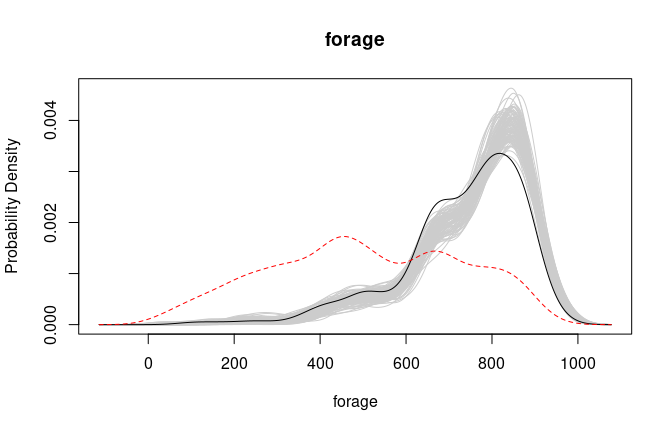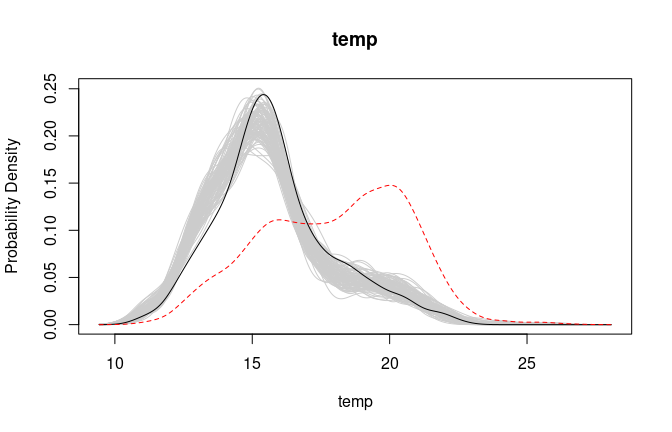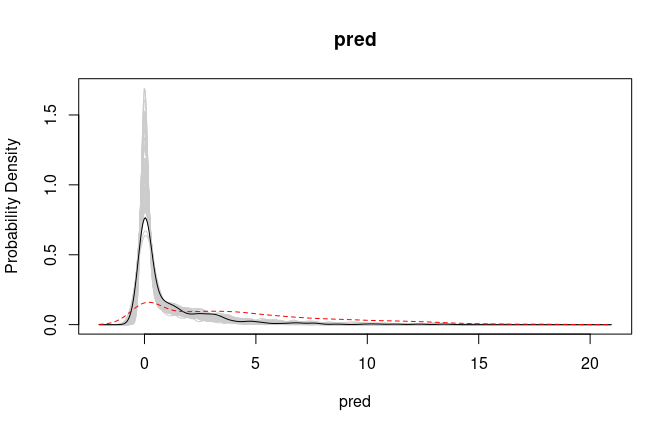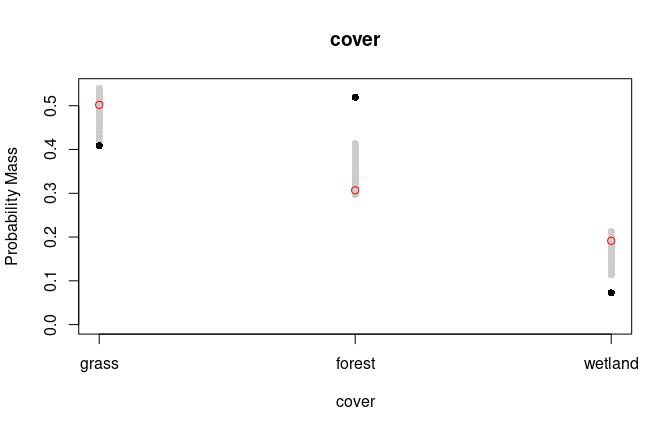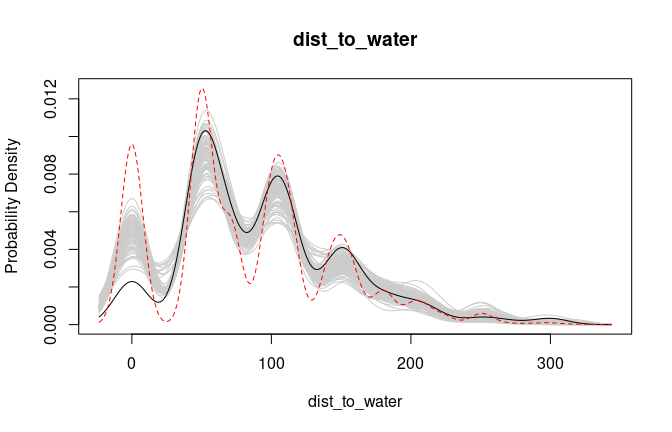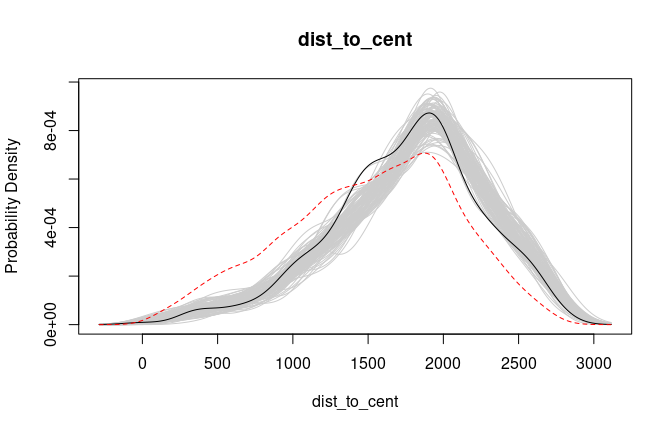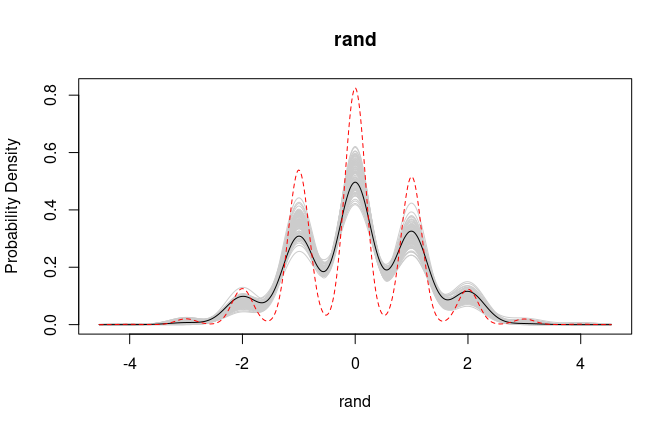Note that the used proportions for `cover == "forest"` and `cover == "wetland"` also fall above and below the modeled envelope, respectively. These are our clues that the model is incorrectly specified.

Note that `dist_to_water` also shows a mismatch between observed and predicted values. If we didn’t already know that this was an unimportant variable, we might consider including it in our model. However, as we will see in the next set of plots, the correct model specification does correctly capture `dist_to_water` without needing to include it in the model.

Now, the right model.

``plot(uhc_hsf_right)``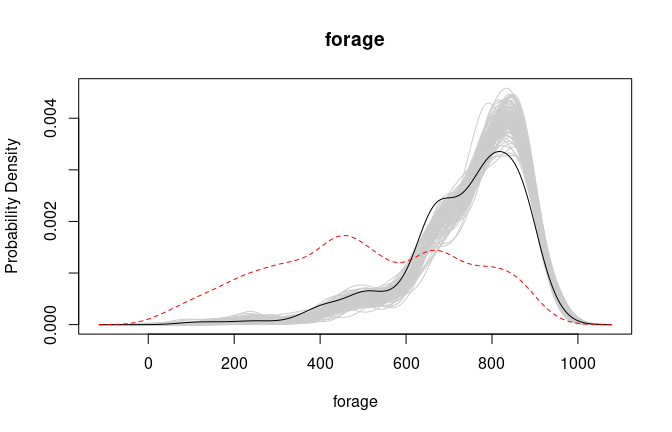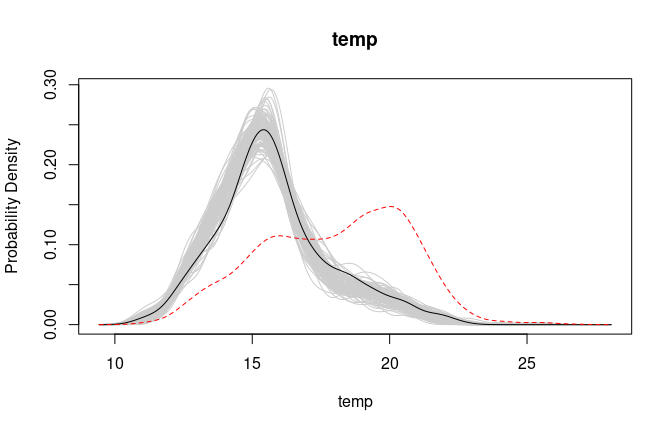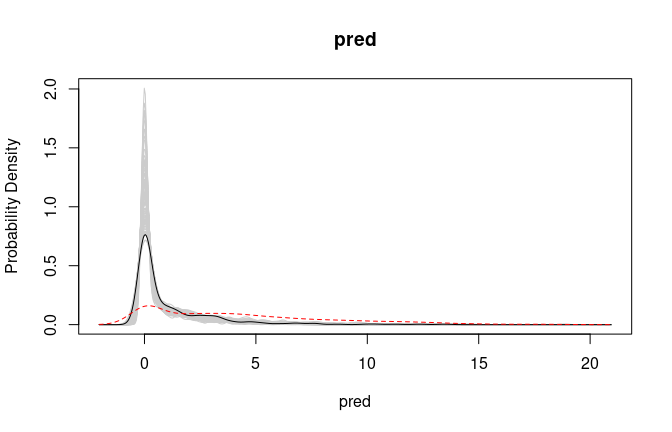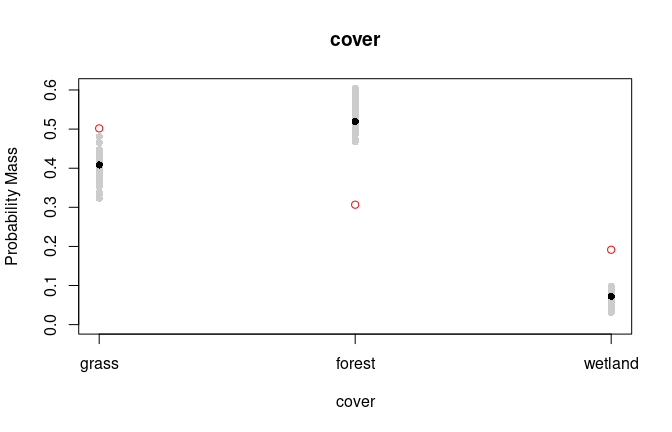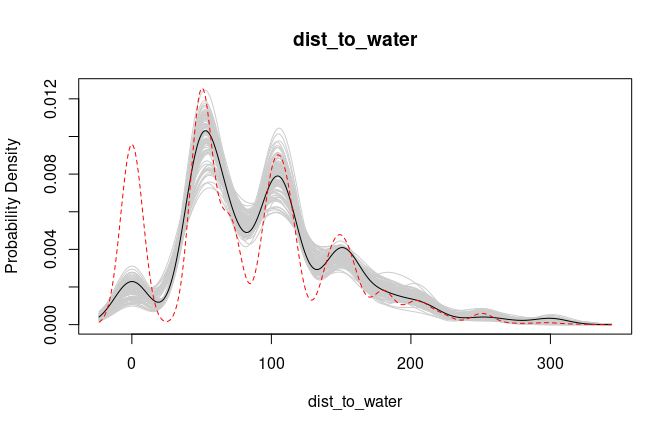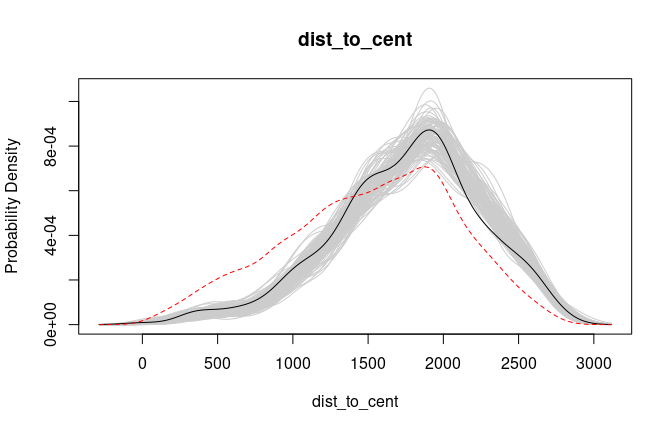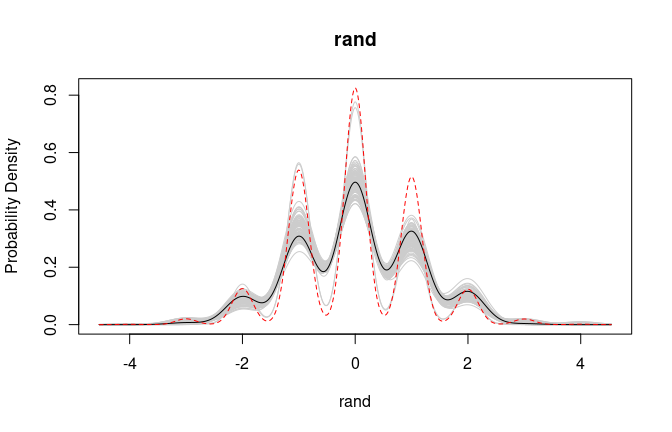We can see these issues have been resolved for our `cover` variables, indicating that our “right” model is, in fact, better calibrated than `hsf_wrong`. Additionally, there is no longer an issue with `dist_to_water`, confirming that we do not need to include it in the model.

Note that with a large number of bootstrap samples, the plots are quite complex and can take multiple seconds to render. If you are working with many candidate variables, this makes examining all the plot quite slow. We have included a few potential solutions to this problem:

• First, you can plot just a single variable, by subsetting the `uhc_data` object by either integers or the names of the variables.
• Second, you can convert the many lines to a single polygon representing a confidence enevelope (with a confidence level of your choice).

Subsetting:

``````# By index
plot(uhc_hsf_right[c(1, 3)])````````````
# By names (vector of >1 names also allowed)
plot(uhc_hsf_right["cover"])``````Envelope:

``````# Coerce to data.frame
df <- as.data.frame(uhc_hsf_right)

# Create confidence envelopes (95% and 100%)
env <- conf_envelope(df, levels = c(0.95, 1))

# Plot
plot(env)``````